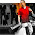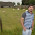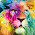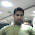### Introduction

'Channels' are used to differentiate different kinds of traffic on radio path. For example, data channels carry users traffic (Youtube, Skype data etc) and control channels carry signaling traffic to manage radio resources, setting up connection etc

The Physical Control Format Indicator Channel (PCFICH) is one of the control channels that works at physical layer. It is used to dynamically indicate the number of symbols to be used for PDCCH.

### Why do we need PCFICH ?

With the help of PCFICH channel, following scenarios are possible:

- Use less symbols for PDCCH if there are a few users with high data rates. Thus leaving more resource elements to be used for user plane data (PDSCH)

- Use more symbols for PDCCH if there are many users with lower data rates e.g VoIP calls in the cell, thus allowing more users capacity.

### Signalled value

PCFICH signalled value depends on channel bandwidth. For channel bandwidth of 3MHz up to 20 Mhz it can carry value of 1, 2 or 3. But for 1.4 Mhz channel bandwidth it can carry value of 2, 3 or 4. Because in case of 1.4 Mhz bandwidth, there are few subcarriers in frequency domain.Therefore, more space is required in time domain to carry PDCCH symbols

### Location of PCFICH symbols on Resource Grid

PCFICH occupies 16 resource elements in frequency domain.  These resource elements are divided into groups of four quadruplets distributed within first OFDMA symbol of each 1 ms subframe. The exact position of PCFICH can be measured from Cell ID and bandwidth using formula given in 3GPP spec 36.211 as below

Where
NRBSC = Number of frequency carriers per Resource block
NDLRB = Number of resource blocks per bandwidth
NcellID  = Physical Cell id

It may look complicated but lets try to understand it with simple example

Lets suppose
Physical Cell id = 20
Bandwidth = 10Mhz  (NDLRB = 50)

Then according to 3GPP Formula

k_Bar = (12/2).(20 mod 2*50) = 6*20 = 120

Then the four PCFICH mapping values are below

120
120 + (50/2)*(12/2) = 270
120 + 2*(50/2)*(12/2)  = 420
120 + 3*(50/2)*(12/2) = 570

Visit LTE Resource Grid generator to validate above mapping values (Enter Cell id = 20 and Bandwidth = 10Mhz)

1.Hi. I really enjoyed my brief visit on your site and I’ll be sure to be back for more.

Thanks!
Kevin
kevincollins1012 gmail.com

1.Hi Kevin, Whats your website ?

2.Thanks!

Cailyn
cailynxxx gmail.com

3.You have explained it very well and in the easier way. I have a question, what elements do those four PCFICH mapping values represent on LTE resource grid ? Like, you have obtained the following mapping values

120
120 + (50/2)*(12/2) = 270
120 + 2*(50/2)*(12/2) = 420
120 + 3*(50/2)*(12/2) = 570

What do they actually represent on resource grid ? is it a 120th subcarrier and 270th subcarrier or what ?? does it mean that 1st REG is located at 120th subcarrier, 2nd REG is located at 270th subcarrier ........ or what ?

4.Thanks for sharing a good explanation.
I just want to ask a question. Does the UE need to check CFI every time when he/she wants to access PDCCH or just make it only first time then after he/she knows it so he/she can use the same CFI later on?

5.I am not able to access link

Thanks
Anek

6.Hello Adnan , I am also having below query-

You have explained it very well and in the easier way. I have a question, what elements do those four PCFICH mapping values represent on LTE resource grid ? Like, you have obtained the following mapping values

120
120 + (50/2)*(12/2) = 270
120 + 2*(50/2)*(12/2) = 420
120 + 3*(50/2)*(12/2) = 570

What do they actually represent on resource grid ? is it a 120th subcarrier and 270th subcarrier or what ?? does it mean that 1st REG is located at 120th subcarrier, 2nd REG is located at 270th subcarrier ........ or what ?

7.Hi,
i m not able to understand this step....
k_Bar = (12/2).(20 mod 2*50) = 6*20 = 120
How 20mod2*50 can come 20?

as per my knowledge 20mode2 is 0 and multiplied by any number will come 0....how 120 is coming?

Thanks,
satyender

8.Satyender, 20 mod 2*50 is 20 mod 100 for which output is 20 which when multiplied by 6 yields 120.

9.The four PCFICH mapping values i.e 120, 270, 420 & 570 represent the sub-carrier IDs in the frequency domain (for 10 MHz bandwidth as mentioned in the example) corresponding to the four REGs where the PCFICH REs are mapped in the 1st symbol of each sub-frame.

1.Hi Srijith...
can you tell where can we find this "sub-carrier IDs"? I mean how UE will come to this "sub-carrier IDs"? And how are they mapped to RE or REGs?

10.PCFICH signalled value depends on channel bandwidth All Vendors. For channel bandwidth of 3MHz up to 20 Mhz it can carry value of 1, 2 or 3. But for 1.4 Mhz channel bandwidth it can carry value of 2, 3 or 4. Because in case of 1.4 Mhz bandwidth All Vendors 2, there are few subcarriers in frequency domain All Vendors 3. Therefore, more space is required in time domain to carry PDCCH symbols

11.1.12.افضل ادوات تنظيف وتعقيم خزانات المياه

تعد الاول افضل شركة تنظيف خزانات بالمدينة المنورة تعمل على استخدام